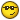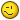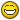# Lisp to insert points from coord from txt## Recommended PostsHello guys,

I'm trying to make a lisp to import points from txt file to autocad, and then to polyline them and to make a union from those points, creating a iregular area. The main purpose is to calculate the area of this created object.

The txt file is something like this:

101 234.442 442.425

102 .....

103....

104...

105...

Anyone there that could help me with this lips ? I kinda need it until tomorow.

##### Share on other sitesQuickest way open file in excel, use space as delimeter.

make a blank line at top put PLINE in cell E1

Then use =concatenate(b2,",",c2) in cell E2

copy the formula all way down as required

you should have

pline

x,y

x,y

x,y etc

Just copy column E and paste to autocad command line.

Yes can do a lisp but this is just as fast.

##### Share on other sitesYou can try this :-

```(defun c:impnt (/ str->list a b c cm d s)
(defun str->list (str / b)
(foreach x (reverse (vl-string->list str))
(cond ((eq x 44) (setq b (cons (list x) b)))
(t
(if (not b)
(setq b (cons (list x) b))
(setq b (cons (cons x (car b)) (cdr b)))
)
)
)
)
(setq b (mapcar '(lambda (x) (vl-list->string (vl-remove 44 x))) b))
(if	(and (> (length b) 1)
)
)
)
(if
(and (setq
a (getfiled "Select CSV File" (getvar "dwgprefix") "txt;csv" 16)
)
(setq s (getdist "\nSpecify Point Size : "))
(setq a (open a "r"))
(setq c (while	(setq b (read-line a))
(setq c (cons (str->list b) c))
)
)
)
(progn
(close a)
(setq cm (getvar 'cmdecho))
(setvar 'cmdecho 0)
(setvar 'pdmode 35)
(setvar 'pdsize s)
c (vl-remove nil c)
)
(foreach	x c
(ssadd	(entmakex (list	(cons 0 "POINT")
(cons 62 3)
(cons 10 x)
)
)
d
)
)
(append	(list (cons 0 "LWPOLYLINE")
(cons 100 "AcDbEntity")
(cons 100 "AcDbPolyline")
(cons 90 (length c))
(cons 70 1)
)
(mapcar (function (lambda (p) (cons 10 p))) c)
)
)
d
)
(command "_.zoom" "_o" d "")
(setvar 'cmdecho cm)
(sssetfirst nil d)
)
)
(princ)
)```

The TXT/CSV format should be like this. Program skips the header automatically.

```Easting (x),Northing (Y),Elevation (Z)
373247.97,2051482.34,0
373271.02,2051446.02,0
373215.57,2051471.86,0
373210.14,2051497.24,0
```

##### Share on other sitessatishrajdev 2 things csv file in 1st post is Ptnum X Y so need to strip off the point number, 2nd is he wants to make a pline of the points so question is do you really need the points ? As you are using "car cadr & caddr" you can drop the "car" which will be the pt number.

As per my post

pline

x,y

x,y

If want both then do a double pass import points then 2nd pass make pline.

##### Share on other sitesYou can try this :-

```(defun c:impnt (/ str->list a b c cm d s)
(defun str->list (str / b)
(foreach x (reverse (vl-string->list str))
(cond ((eq x 44) (setq b (cons (list x) b)))
(t
(if (not b)
(setq b (cons (list x) b))
(setq b (cons (cons x (car b)) (cdr b)))
)
)
)
)
(setq b (mapcar '(lambda (x) (vl-list->string (vl-remove 44 x))) b))
(if	(and (> (length b) 1)
)
)
)
(if
(and (setq
a (getfiled "Select CSV File" (getvar "dwgprefix") "txt;csv" 16)
)
(setq s (getdist "\nSpecify Point Size : "))
(setq a (open a "r"))
(setq c (while	(setq b (read-line a))
(setq c (cons (str->list b) c))
)
)
)
(progn
(close a)
(setq cm (getvar 'cmdecho))
(setvar 'cmdecho 0)
(setvar 'pdmode 35)
(setvar 'pdsize s)
c (vl-remove nil c)
)
(foreach	x c
(ssadd	(entmakex (list	(cons 0 "POINT")
(cons 62 3)
(cons 10 x)
)
)
d
)
)
(append	(list (cons 0 "LWPOLYLINE")
(cons 100 "AcDbEntity")
(cons 100 "AcDbPolyline")
(cons 90 (length c))
(cons 70 1)
)
(mapcar (function (lambda (p) (cons 10 p))) c)
)
)
d
)
(command "_.zoom" "_o" d "")
(setvar 'cmdecho cm)
(sssetfirst nil d)
)
)
(princ)
)```

The TXT/CSV format should be like this. Program skips the header automatically.

```Easting (x),Northing (Y),Elevation (Z)
373247.97,2051482.34,0
373271.02,2051446.02,0
373215.57,2051471.86,0
373210.14,2051497.24,0
```

Thanks for that awesome help. I'm trying to make it on education purpose for my faculty.

I will check it out but untill now it worked perfectly.

have a nice day.

##### Share on other sites1st post is Ptnum X Y so need to strip off the point number

I know, I wrote this routine for someone and posted it directly. I have modified following code as per OP's requirement.

```(defun c:impnt (/ str->list a b c cm d s)
(defun str->list (str / b)
(foreach x (reverse (vl-string->list str))
(cond ((eq x 44) (setq b (cons (list x) b)))
(t
(if (not b)
(setq b (cons (list x) b))
(setq b (cons (cons x (car b)) (cdr b)))
)
)
)
)
(setq b (mapcar '(lambda (x) (vl-list->string (vl-remove 44 x))) b))
(if	(and (> (length b) 1)
)
)
)
(if
(and (setq
a (getfiled "Select CSV File" (getvar "dwgprefix") "txt;csv" 16)
)
(setq s (getdist "\nSpecify Point Size : "))
(setq a (open a "r"))
(setq c (while	(setq b (read-line a))
(setq c (cons (str->list b) c))
)
)
)
(progn
(close a)
(setq cm (getvar 'cmdecho))
(setvar 'cmdecho 0)
(setvar 'pdmode 35)
(setvar 'pdsize s)
c (vl-remove nil c)
)
(foreach	x c
(ssadd	(entmakex (list	(cons 0 "POINT")
(cons 62 3)
(cons 10 x)
)
)
d
)
)
(append	(list (cons 0 "LWPOLYLINE")
(cons 100 "AcDbEntity")
(cons 100 "AcDbPolyline")
(cons 90 (length c))
(cons 70 1)
)
(mapcar (function (lambda (p) (cons 10 p))) c)
)
)
d
)
(command "_.zoom" "_o" d "")
(setvar 'cmdecho cm)
(sssetfirst nil d)
)
)
(princ)
)```

Another one without point entities:

```(defun c:impnt (/ str->list a c cm d)
(defun str->list (str / b)
(foreach x (reverse (vl-string->list str))
(cond ((eq x 44) (setq b (cons (list x) b)))
(t
(if (not b)
(setq b (cons (list x) b))
(setq b (cons (cons x (car b)) (cdr b)))
)
)
)
)
(setq b (mapcar '(lambda (x) (vl-list->string (vl-remove 44 x))) b))
(if	(and (> (length b) 1)
)
)
)
(if
(and (setq
a (getfiled "Select CSV File"
(getvar "dwgprefix")
"txt;csv"
16
)
)
(setq a (open a "r"))
(setq c (while	(setq b (read-line a))
(setq c (cons (str->list b) c))
)
)
)
(progn
(close a)
(setq cm (getvar 'cmdecho))
(setvar 'cmdecho 0)
c (vl-remove nil c)
)
(append	(list (cons 0 "LWPOLYLINE")
(cons 100 "AcDbEntity")
(cons 100 "AcDbPolyline")
(cons 90 (length c))
(cons 70 1)
)
(mapcar (function (lambda (p) (cons 10 p))) c)
)
)
d
)
(command "_.zoom" "_o" d "")
(setvar 'cmdecho cm)
(sssetfirst nil d)
)
)
(princ)
)```

##### Share on other sitesThanks for that awesome help. I'm trying to make it on education purpose for my faculty.

I will check it out but untill now it worked perfectly.

have a nice day.

You're welcome:)

##### Share on other sitesHi Kr1stal1 . I use this lisp for this job .Support may option for files.

http://www.hawsedc.com/gnu/pointsin.php

##### Share on other sitesSatishrajdev thats the problem with survey csv files they can be all over the place in CIV3D you have styles so nominate how the csv looks P,x,y,z,d etc.

The import a csv and string it based on codes is still on my list to do one day.

##### Share on other sites.. P,x,y,z,d etc.

The import a csv and string it based on codes is still on my list to do one day.1+

```[color="green"]..
...[/color]
```

vlisp suggestion vl-every

`[color="green"] (list (atof (cadr b)) (atof (caddr b)) (atof (cadddr b)))[/color]`

FYI try mapcar##### Share on other sitesThanks everyone for the help.## Join the conversation

You can post now and register later. If you have an account, sign in now to post with your account.
Note: Your post will require moderator approval before it will be visible.×   Pasted as rich text.   Restore formatting

Only 75 emoji are allowed.